Next: Spherical Wave Expansion of Up: Multipole Expansion Previous: Sources of Multipole Radiation

# Radiation from Linear Centre-Fed Antenna

As an illustration of the use of a multipole expansion for a source whose dimensions are comparable to a wavelength, consider the radiation from a linear centre-fed antenna. We assume that the antenna runs along the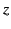-axis, and extends from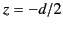to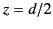. The current flowing along the antenna vanishes at the end points, and is an even function of. Thus, we can write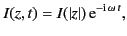(1547)

where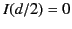. Because the current flows radially,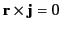. Furthermore, there is no intrinsic magnetization. Thus, according to Equations (1547)-(1548), all of the magnetic multipole coefficients,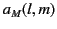, vanish. In order to calculate the electric multipole coefficients,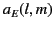, we need expressions for the charge and current densities. In spherical polar coordinates, the current density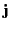can be written in the form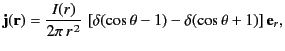(1548)

for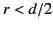, where the delta functions cause the current to flow only upwards and downwards along the-axis. From the continuity equation (1522), the charge density is given by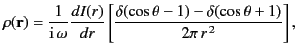(1549)

for.

The above expressions forand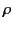can be substituted into Equation (1543) to give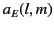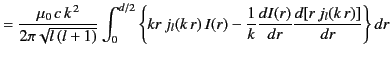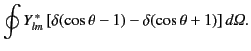(1550)

The angular integral has the value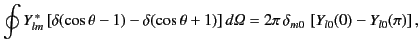(1551)

showing that only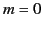multipoles are generated. This is hardly surprising, given the cylindrical symmetry of the antenna. Thespherical harmonics are even (odd) about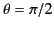for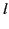even (odd). Hence, the only nonvanishing multipoles haveodd, and the angular integral reduces to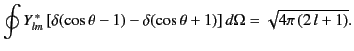(1552)

After some slight rearrangement, Equation (1552) can be written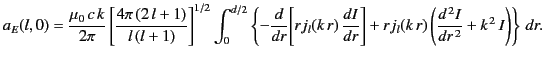(1553)

In order to evaluate the above integral, we need to specify the current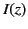along the antenna. In the absence of radiation, the sinusoidal time variation at frequency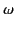implies a sinusoidal space variation with wavenumber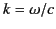. However, the emission of radiation generally modifies the current distribution. The correct currentcan only be found be solving a complicated boundary value problem. For the sake of simplicity, we assume thatis a known function: specifically,(1554)

for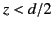, where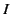is the peak current. With a sinusoidal current, the second term in curly brackets in Equation (1555) vanishes. The first term is a perfect differential. Consequently, Equations (1555) and (1556) yield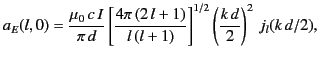(1555)

forodd.

Let us consider the special cases of a half-wave antenna (i.e.,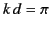, so that the length of the antenna is half a wavelength) and a full-wave antenna (i.e.,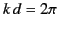). For these two values of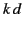, the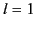electric multipole coefficient is tabulated in Table 3, along with the relative values for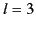and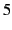. It is clear, from the table, that the coefficients decrease rapidly in magnitude asincreases, and that highercoefficients are more important the larger the source dimensions. However, even for a full-wave antenna, it is generally sufficient to retain only theandcoefficients when calculating the angular distribution of the radiation. It is certainly adequate to keep only these two harmonics when calculating the total radiated power (which depends on the sum of the squares of the coefficients).

Table 3: The first few electric multipole coefficients for a half-wave and a full-wave antenna.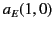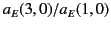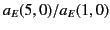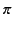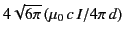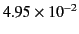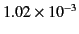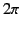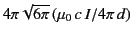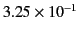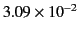In the radiation zone, the multipole fields (1479)-(1480) reduce to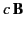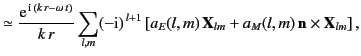(1556)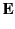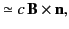(1557)

where use has been made of the asymptotic form (1432). The time-averaged power radiated per unit solid angle is given by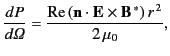(1558)

or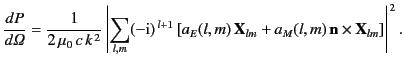(1559)

Retaining only theandelectric multipole coefficients, the angular distribution of the radiation from the antenna takes the form(1560)

where use has been made of Equation (1476). The various factors in the absolute square are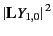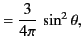(1561)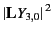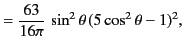(1562)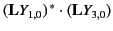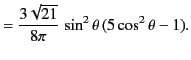(1563)

With these angular factors, Equation (1561) becomes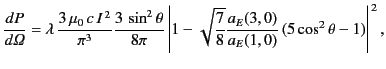(1564)

where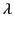equals 1 for a half-wave antenna, and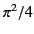for a full-wave antenna. The coefficient in front of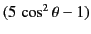is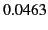and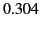for the half-wave and full-wave antenna, respectively. It turns out that the radiation pattern obtained from the two-term multipole expansion specified in the previous equation is almost indistinguishable from the exact result for the case of a half-wave antenna. For the case of a full-wave antenna, the two-term expansion yields a radiation pattern that differs from the exact result by less than 5 percent.

The total power radiated by the antenna is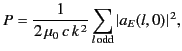(1565)

where use has been made of Equation (1500). It is evident from Table 3 that a two-term multipole expansion gives an accurate expression for the power radiated by both a half-wave and a full-wave antenna. In fact, a one-term multipole expansion gives a fairly accurate result for the case of a half-wave antenna.

It is clear, from the previous analysis, that the multipole expansion converges rapidly when the source dimensions are of order the wavelength of the radiation. It is also clear that if the source dimensions are much less than the wavelength then the multipole expansion is likely to be completely dominated by the term corresponding to the lowest value of.Next: Spherical Wave Expansion of Up: Multipole Expansion Previous: Sources of Multipole Radiation
Richard Fitzpatrick 2014-06-27